# 深度学习LSTM网络：自然语言处理实践

NLP 自学习平台，3个模型定制额度 1个月
NLP自然语言处理_基础版，每接口每天50万次
NLP自然语言处理_高级版，每接口累计50万次

+关注继续查看

## 1 RNN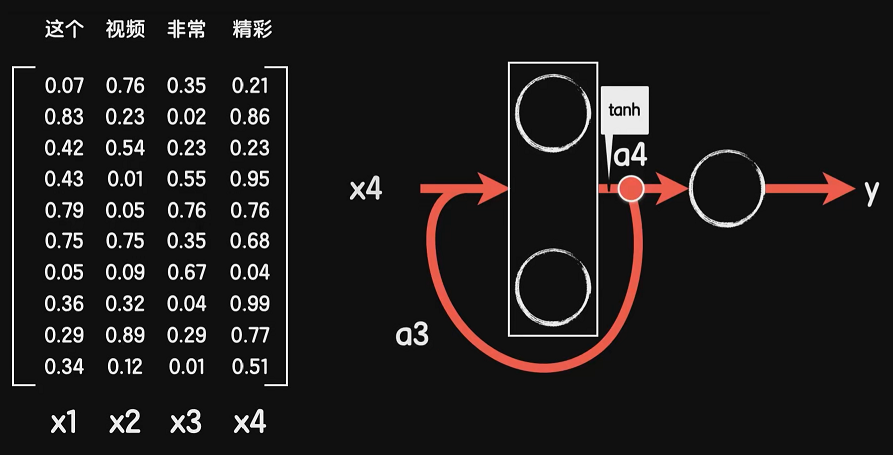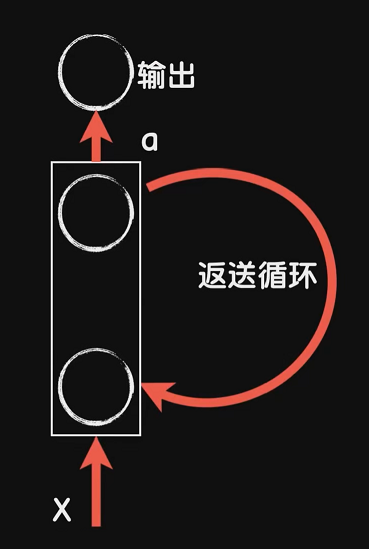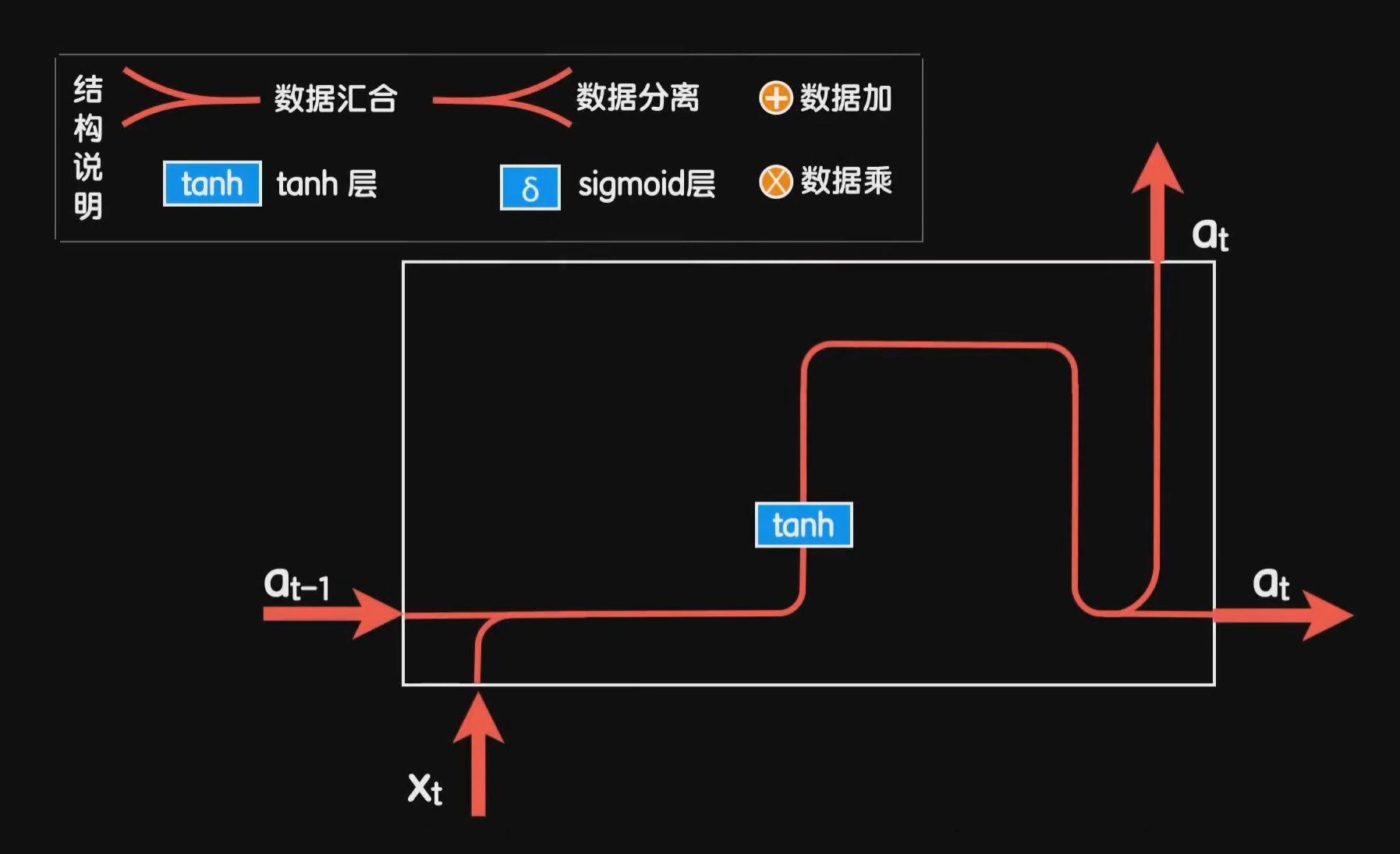LSTM结构中的输出再次经过一个tanh函数，而原先的输出则变成了一个叫细胞状态的东西，这个细胞状态就是LSTM结构能应对长依赖问题的关键，这个结构的输入相应的变成了上一个细胞状态和上一步的输出。为了实现记忆和遗忘，LSTM结构使用了两个门来实现：遗忘门、更新门。最终的输出也有一个输出门，根据输出的内容的重要性进行强弱输出。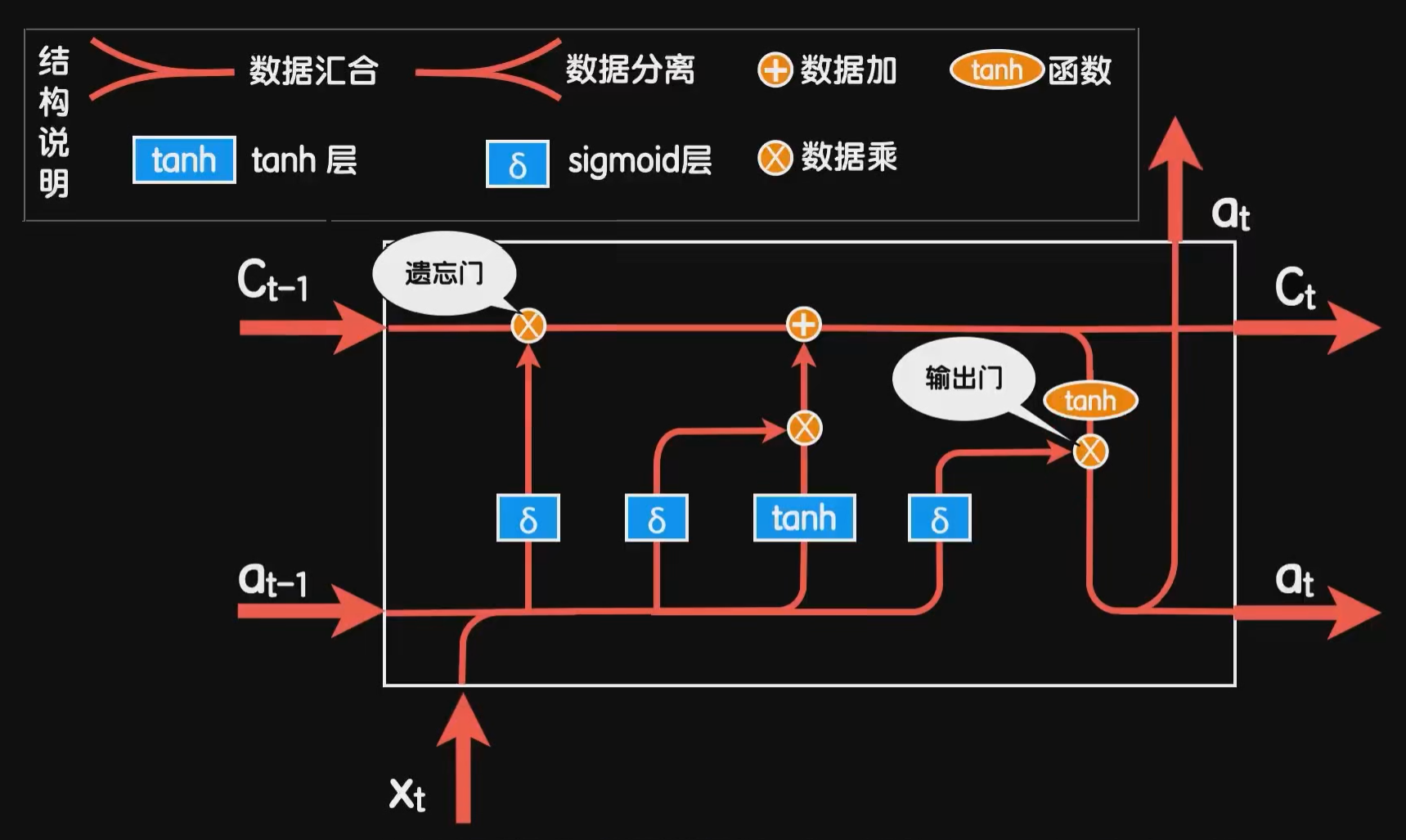🔗有关LSTM的更多理解，可以参考这篇博客：《Understanding LSTM Networks》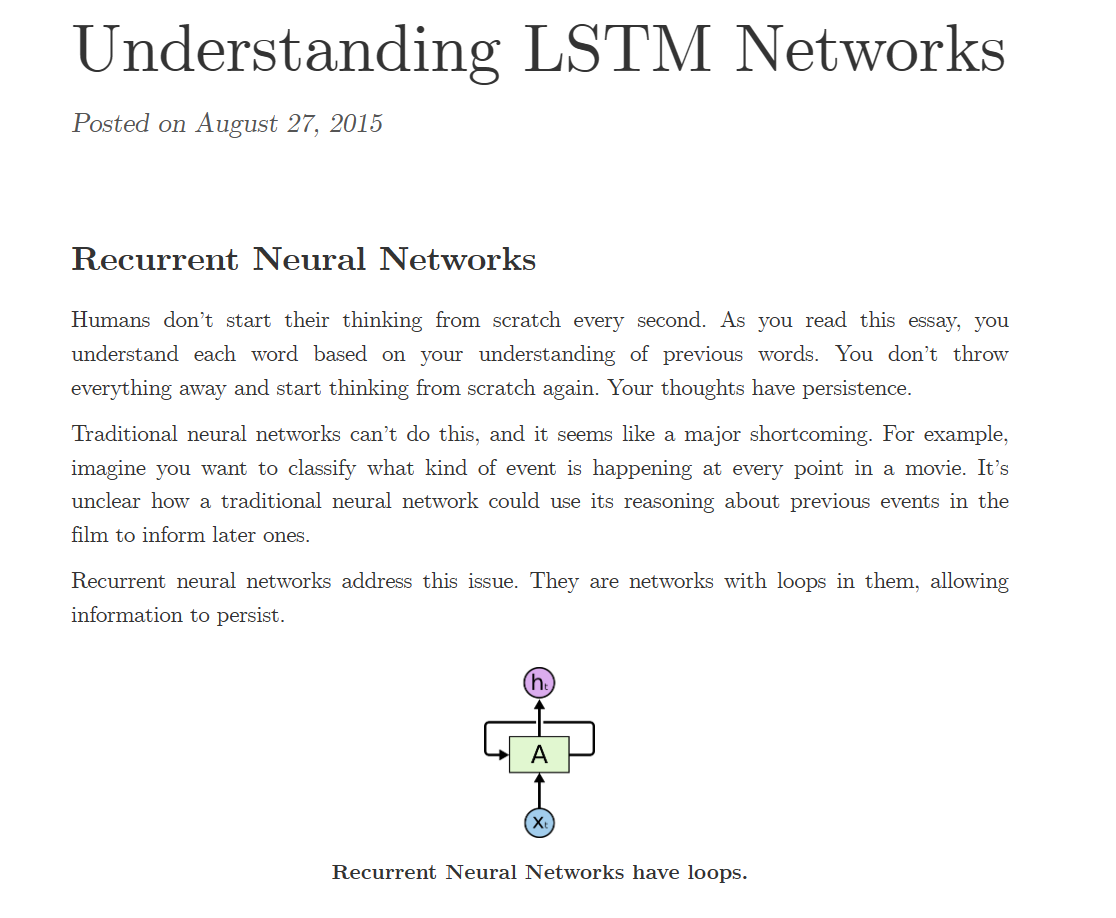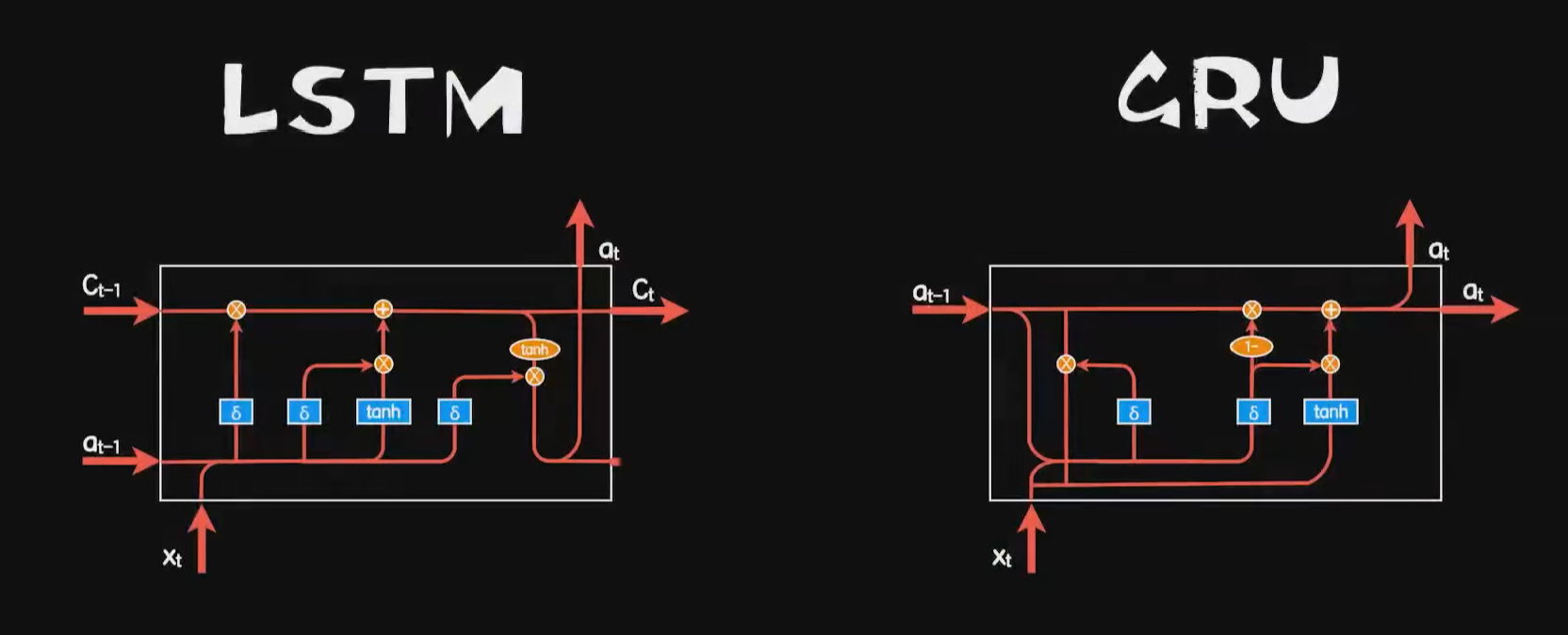## 2 编程实验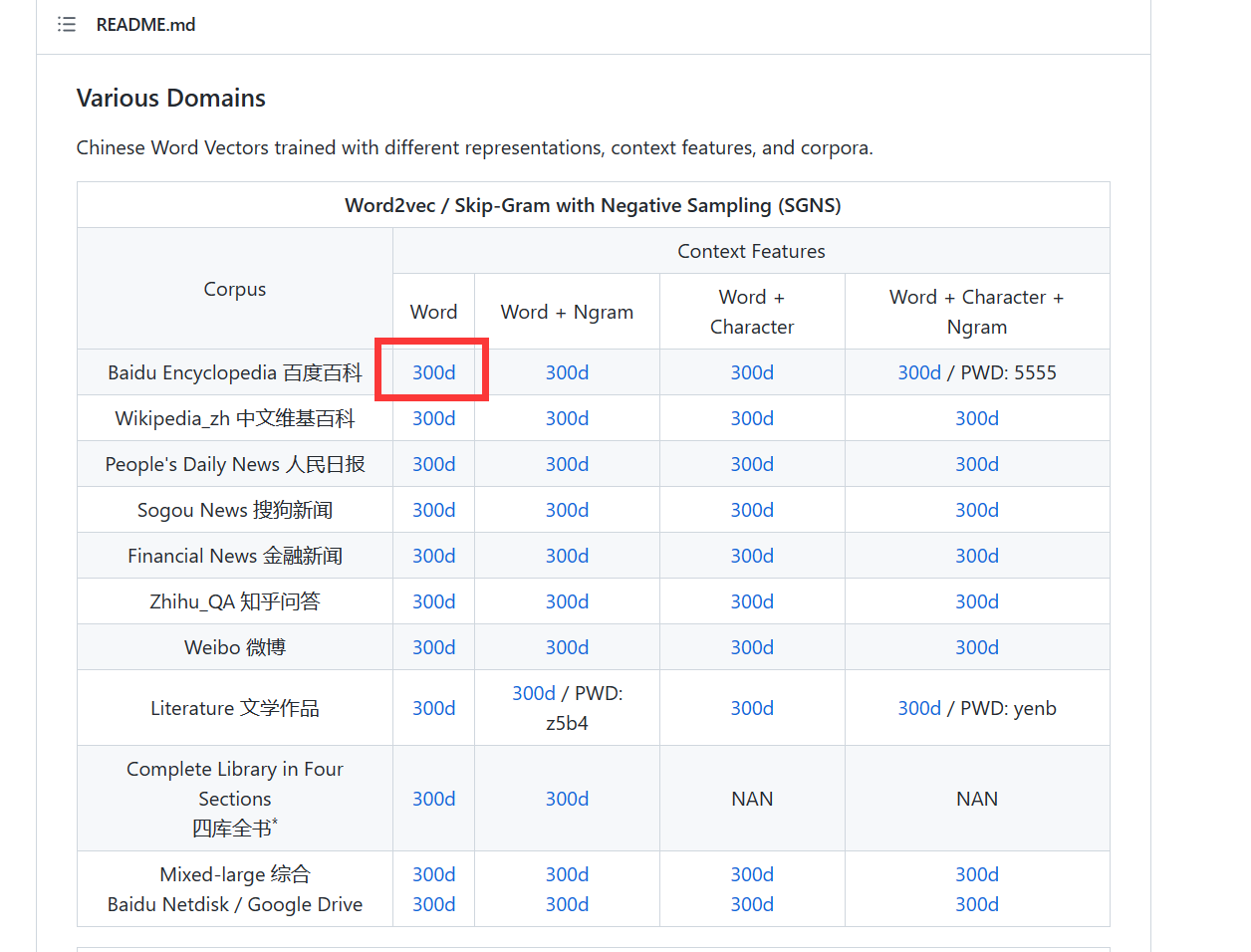import os
import numpy as np

embeddings_index = {}
f = open(os.path.dirname(os.path.abspath(__file__)) + '/sgns.target.word-word.dynwin5.thr10.neg5.dim300.iter5',
encoding='utf8')
for line in f:
values = line.split()
word = values
coefs = np.asarray(values[1:], dtype='float32')
embeddings_index[word] = coefs
f.close()

print('Found %s word vectors.' % len(embeddings_index))
return embeddings_index


cat,label,review

...



import os
import keras
import numpy as np
import keras.preprocessing.text as text
import re
import jieba
import random

xs = []
ys = []
with open(os.path.dirname(os.path.abspath(__file__))+'/online_shopping_10_cats.csv','r',encoding='utf-8') as f:
while line:
if not line:
break
contents = line.split(',')

# if contents=="书籍":
#   continue

label = int(contents)
review = contents
if len(review)>20:
continue

xs.append(review)
ys.append(label)

xs = np.array(xs)
ys = np.array(ys)

#打乱数据集
indies = [i for i in range(len(xs))]
random.seed(666)
random.shuffle(indies)
xs = xs[indies]
ys = ys[indies]

m = len(xs)
cutpoint = int(m*4/5)
x_train = xs[:cutpoint]
y_train = ys[:cutpoint]

x_test = xs[cutpoint:]
y_test = ys[cutpoint:]

print('总样本数量:%d' % (len(xs)))
print('训练集数量:%d' % (len(x_train)))
print('测试集数量:%d' % (len(x_test)))

return x_train,y_train,x_test,y_test

def createWordIndex(x_train,x_test):
x_all = np.concatenate((x_train,x_test),axis=0)
#建立词索引
tokenizer = text.Tokenizer()
#create word index
word_dic = {}
voca = []
for sentence in x_all:
# 去掉标点
sentence = re.sub("[\s+\.\!\/_,$%^*(+\"\']+|[+——！，。？、~@#￥%……&*（）]+", "", sentence) # 结巴分词 cut = jieba.cut(sentence) #cut_list = [ i for i in cut ] for word in cut: if not (word in word_dic): word_dic[word]=0 else: word_dic[word] +=1 voca.append(word) word_dic = sorted(word_dic.items(), key = lambda kv:kv,reverse=True) voca = [v for v in word_dic] tokenizer.fit_on_texts(voca) print("voca:"+str(len(voca))) return len(voca),tokenizer.word_index def word2Index(words,word_index): vecs = [] for sentence in words: # 去掉标点 sentence = re.sub("[\s+\.\!\/_,$%^*(+\"\']+|[+——！，。？、~@#￥%……&*（）]+", "", sentence)
# 结巴分词
cut = jieba.cut(sentence)
#cut_list = [ i for i in cut ]
index=[]

for word in cut:
if word in word_index:
index.append(float(word_index[word]))

# if len(index)>25:
#   index = index[0:25]
vecs.append(np.array(index))

return np.array(vecs)


import shopping_data
# 数据对齐

from keras.models import Sequential
from keras.layers import Dense, Embedding
# 导入LSTM
from keras.layers import LSTM
# 读取中文词向量工具
import chinese_vec
import numpy as np

x_train, y_train, x_test, y_test = shopping_data.load_data()
# 打印数据集
# print('x_train.shape：', x_train.shape)
# print('y_train.shape：', y_train.shape)
# print('x_test.shape：', x_test.shape)
# print('y_test.shape：', y_test.shape)
# print(x_train)
# print(y_train)

vocalen, word_index = shopping_data.createWordIndex(x_train, x_test)
# print(word_index)
# print('词典总词数：', vocalen)

# 转化为索引向量
x_train_index = shopping_data.word2Index(x_train, word_index)
x_test_index = shopping_data.word2Index(x_test, word_index)
# 每一句话的索引向量个数不一样，我们需要把序列按照maxlen对齐
maxlen = 25

# 自行构造词嵌入矩阵
embedding_matrix = np.zeros((vocalen, 300))

for word, i in word_index.items():
embedding_vector = word_vecs.get(word)
if embedding_vector is not None:
embedding_matrix[i] = embedding_vector

# 神经网络模型
model = Sequential()
Embedding(
trainable=False, # 冻结这一层，不要让它预训练
weights=[embedding_matrix],
input_dim=vocalen, # 输入维度
output_dim=300, # 输出维度
input_length=maxlen # 序列长度
)
)
128, # 输出数据的维度
return_sequences=True # 每一个都输出结果
))

# 二分类问题，使用sigmoid激活函数
model.compile(
loss='binary_crossentropy', # 适用于二分类问题的交叉熵代价函数
metrics=['accuracy']
)
# 训练
model.fit(x_train_index, y_train, batch_size=512, epochs=200)
score, acc = model.evaluate(x_test_index, y_test)
# 评估
print('Test score:', score)
print('Test accuracy:', acc)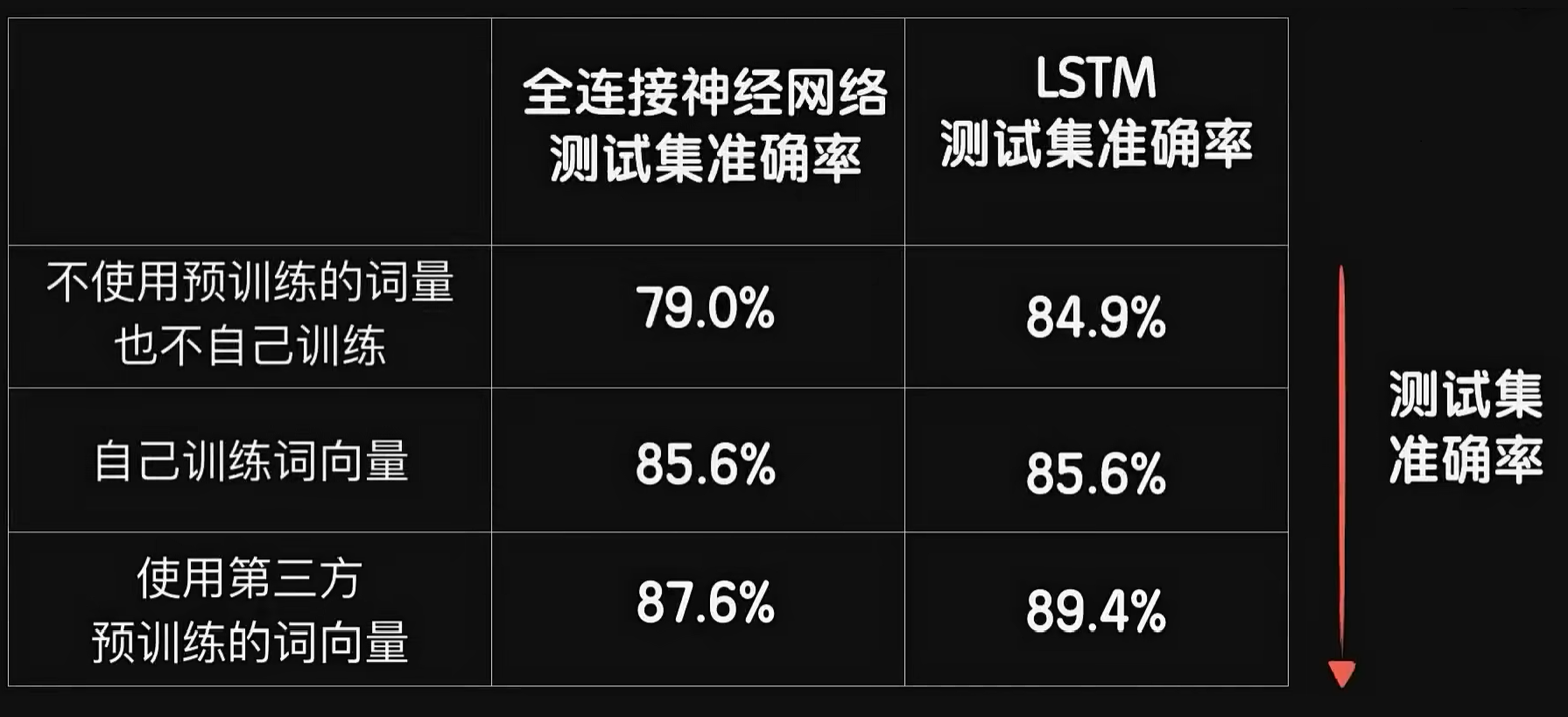|
2天前
|

29 1
|
9天前
|

14 0
|
14天前
|

14 0
|
14天前
|

17 0
|
19天前
|

11 0
|
20天前
|

12 0
|
23天前
|

10 0
|
28天前
|

32 0
|
29天前
|

20 0
|
30天前
|

61 1